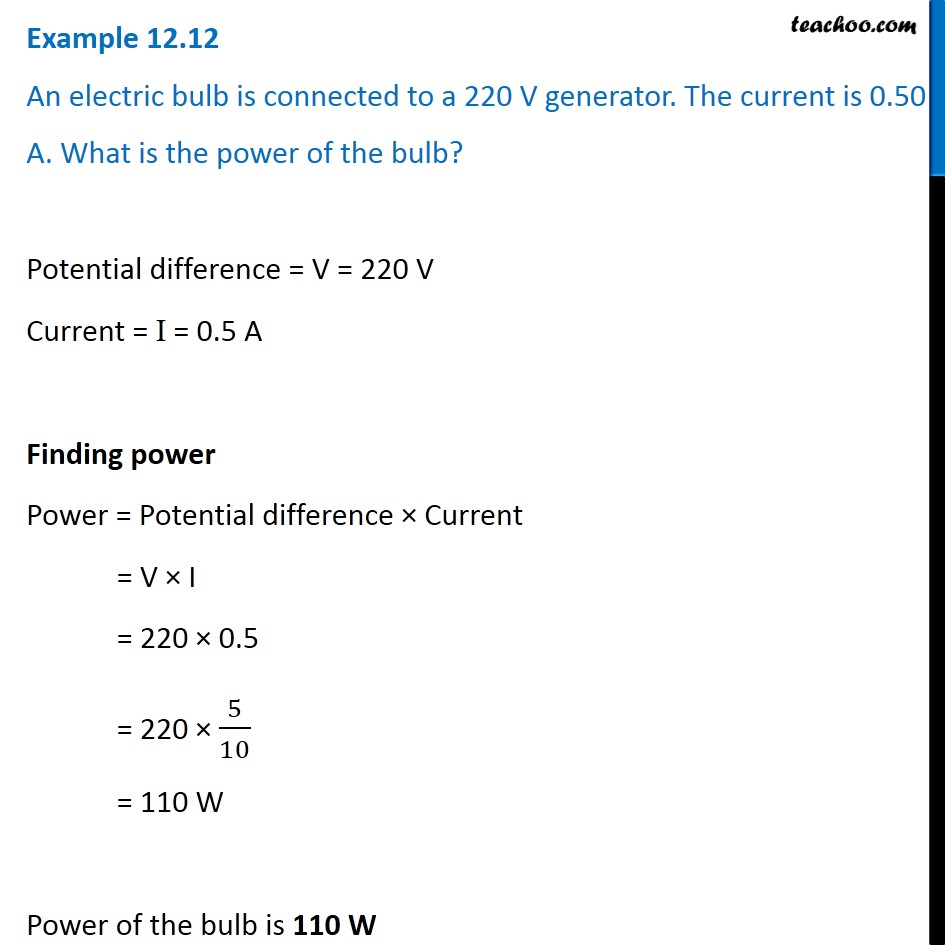Examples from NCERT Book

Class 10
Chapter 12 Class 10 - Electricity

## An electric bulb is connected to a 220 V generator. The current is 0.50 A. What is the power of the bulb?Learn in your speed, with individual attention - Teachoo Maths 1-on-1 Class

### Transcript

Example 12.12 An electric bulb is connected to a 220 V generator. The current is 0.50 A. What is the power of the bulb? Potential difference = V = 220 V Current = I = 0.5 A Finding power Power = Potential difference × Current = V × I = 220 × 0.5 = 220 × 5/10 = 110 W Power of the bulb is 110 W